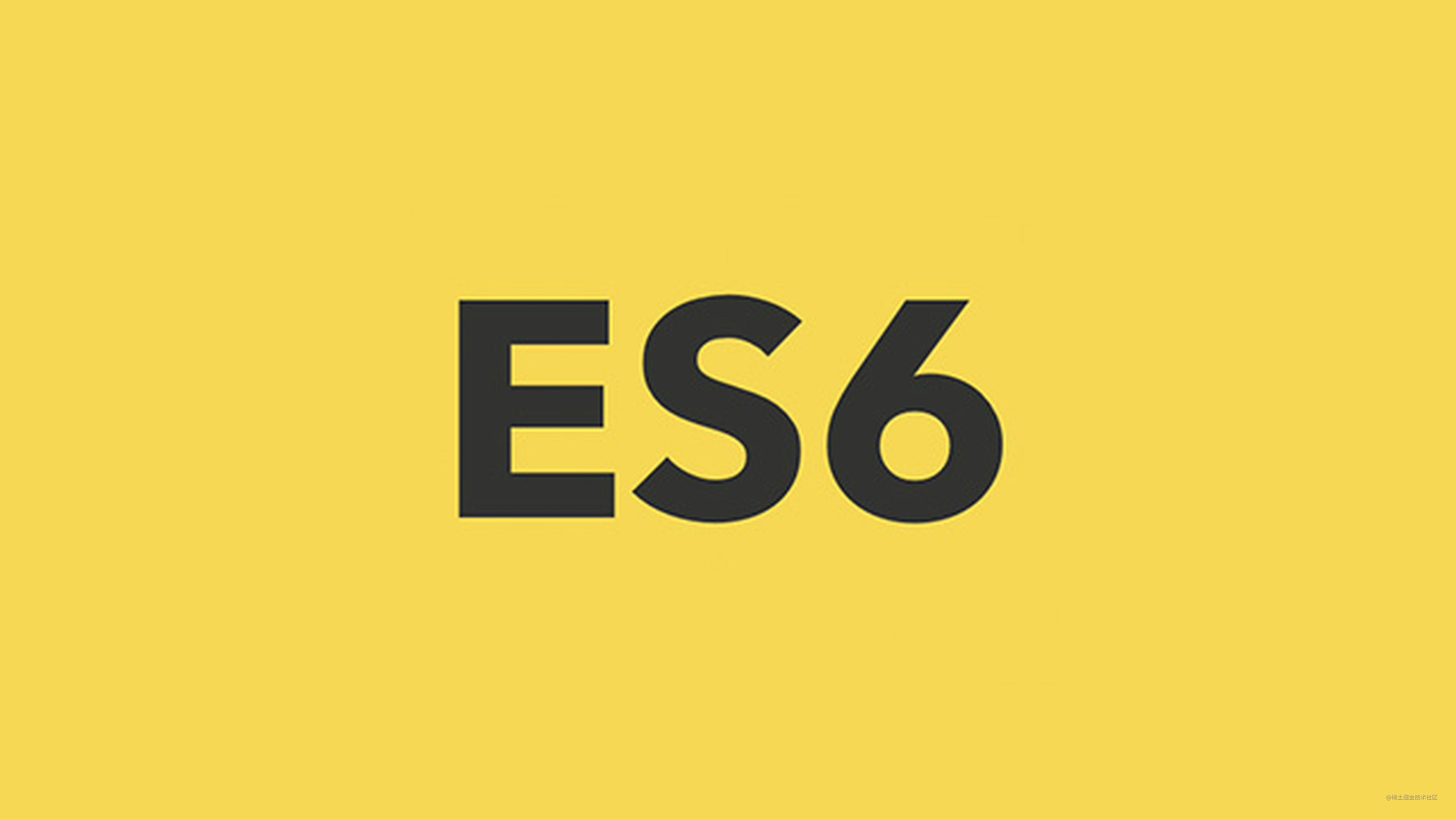# ES6 系列之箭头函数## 回顾

ES6 增加了箭头函数：

``````let func = value => value;

``````let func = function (value) {
return value;
};

``````let func = (value, num) => value * num;

``````let func = (value, num) => {
return value * num
};

``````let func = (value, num) => ({total: value * num});

``````let func = ({value, num}) => ({total: value * num})

// 使用
var result = func({
value: 10,
num: 10
})

console.log(result); // {total: 100}

``````handleEvent = () => {
this.setState({
data: this.state.data.set("key", "value")
})
};

``````handleEvent = () => {
this.setState(({data}) => ({
data: data.set("key", "value")
}))
};

## 比较

### 1.没有 this

``````// 传入元素 id 值即可绑定该元素点击时改变背景色的事件
new Button("button")

HTML 代码如下：

``````<button id="button">点击变色</button>

JavaScript 代码如下：

``````function Button(id) {
this.element = document.querySelector("#" + id);
this.bindEvent();
}

Button.prototype.bindEvent = function() {
};

Button.prototype.setBgColor = function() {
this.element.style.backgroundColor = '#1abc9c'
};

var button = new Button("button");

``````Button.prototype.setBgColor = function() {
this.style.backgroundColor = '#1abc9c'
};

``````Button.prototype.setBgColor = function() {
this.setElementColor();
this.setOtherElementColor();
};

``````Button.prototype.bindEvent = function() {
};

``````Button.prototype.bindEvent = function() {
this.element.addEventListener("click", event => this.setBgColor(event), false);
};

``````var value = 1;
var result = (() => this.value).bind({value: 2})();
console.log(result); // 1

### 2. 没有 arguments

``````function constant() {
return () => arguments
}

var result = constant(1);
console.log(result()); // 1

``````let nums = (...nums) => nums;

### 3. 不能通过 new 关键字调用

JavaScript 函数有两个内部方法：[[Call]] 和 [[Construct]]。

``````var Foo = () => {};
var foo = new Foo(); // TypeError: Foo is not a constructor

### 5. 没有原型

``````var Foo = () => {};
console.log(Foo.prototype); // undefined

## 总结

An arrow function expression has a shorter syntax than a function expression and does not have its own this, arguments, super, or new.target. These function expressions are best suited for non-method functions, and they cannot be used as constructors.

A method is a function which is a property of an object.

``````var obj = {
i: 10,
b: () => console.log(this.i, this),
c: function() {
console.log( this.i, this)
}
}
obj.b();
// undefined Window
obj.c();
// 10, Object {...}

## 自执行函数

``````(function(){
console.log(1)
})()

``````(function(){
console.log(1)
}())

``````(() => {
console.log(1)
})()

``````(() => {
console.log(1)
}())

## ES6 系列

ES6 系列目录地址：github.com/mqyqingfeng…

ES6 系列预计写二十篇左右，旨在加深 ES6 部分知识点的理解，重点讲解块级作用域、标签模板、箭头函数、Symbol、Set、Map 以及 Promise 的模拟实现、模块加载方案、异步处理等内容。